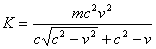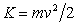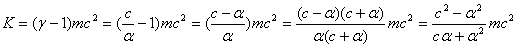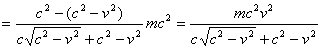Home English Hebrew

 The Millennium Relativity Kinetic Energy   Rafi Moor     The Millennium Theory of Relativity suggests a new expression for the kinetic energy and say it is better then the one proposed by Einstein. The new equation is this:This equation is just another form of the known Einstein's equation. It is true that there are some advantages to presenting kinetic energy in this form: It can be practically used for all the range of velocities and it reduces to the non-relativistic equationwhen v  <<  c, but this is not a new equation. The difference that is shown in the Millennium Relativity between the results of the two equations is just a precision problem. The fact that the classic equation is built as a subtraction of two elements that gets very close when v  <<  c makes it impractical to use at low speeds. But mathematically there is no difference between the two. The new form can be directly derived from the classic equation as shown below:   For convenience we define:(1)   The Lorenz factor can now be written as:(2)       We start from the classic equation and end up with the millennium equation:We now substitute (1)